# Colleges with the lowest SAT scores in Iowa

Top 10 colleges in Iowa with the lowest SAT scores
Looking for the colleges with the lowest SAT scores in Iowa? Well you're in luck! We've compiled a national college database and have created a list of the top 10 universities with the lowest SAT scores in Iowa below. If you are not a good test taker or worried about your test scores, this list is for you. These are the schools whose applicants had the lowest average SAT scores in Iowa, which means that you can get into these colleges with a lower SAT score. We also include each college's ACT scores and acceptance rate so that you can see where you would have the easiest time getting in. Read on to find out more.

## Waldorf College SAT scores

The average SAT score for Waldorf College is 1030.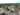The average SAT score of 1030 breaks down into:

• SAT math: 510

The average ACT score for Waldorf College is 21 and their acceptance rate is 71.9%.

## Upper Iowa University SAT scores

The average SAT score for Upper Iowa University is 1050.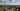The average SAT score of 1050 breaks down into:

• SAT math: 520

The average ACT score for Upper Iowa University is 21 and their acceptance rate is 52.2%.

## Wartburg College SAT scores

The average SAT score for Wartburg College is 1090.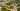The average SAT score of 1090 breaks down into:

• SAT math: 550

The average ACT score for Wartburg College is 23 and their acceptance rate is 75.7%.

## Loras College SAT scores

The average SAT score for Loras College is 1099.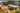The average SAT score of 1099 breaks down into:

• SAT math: 560

The average ACT score for Loras College is 23 and their acceptance rate is 92.7%.

## Central College SAT scores

The average SAT score for Central College is 1110.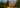The average SAT score of 1110 breaks down into:

• SAT math: 530

The average ACT score for Central College is 24 and their acceptance rate is 64.3%.

## Iowa Wesleyan College SAT scores

The average SAT score for Iowa Wesleyan College is 1110.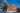The average SAT score of 1110 breaks down into:

• SAT math: 560

The average ACT score for Iowa Wesleyan College is 21 and their acceptance rate is 41.3%.

## Saint Ambrose University SAT scores

The average SAT score for Saint Ambrose University is 1140.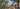The average SAT score of 1140 breaks down into:

• SAT math: 570

The average ACT score for Saint Ambrose University is 23 and their acceptance rate is 60.6%.

## University of Northern Iowa SAT scores

The average SAT score for University of Northern Iowa is 1140.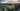The average SAT score of 1140 breaks down into:

• SAT math: 570

The average ACT score for University of Northern Iowa is 23 and their acceptance rate is 80.7%.

## Luther College SAT scores

The average SAT score for Luther College is 1165.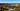The average SAT score of 1165 breaks down into:

• SAT math: 590

The average ACT score for Luther College is 26 and their acceptance rate is 65.1%.

## Simpson College SAT scores

The average SAT score for Simpson College is 1180.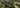The average SAT score of 1180 breaks down into:

• SAT math: 590